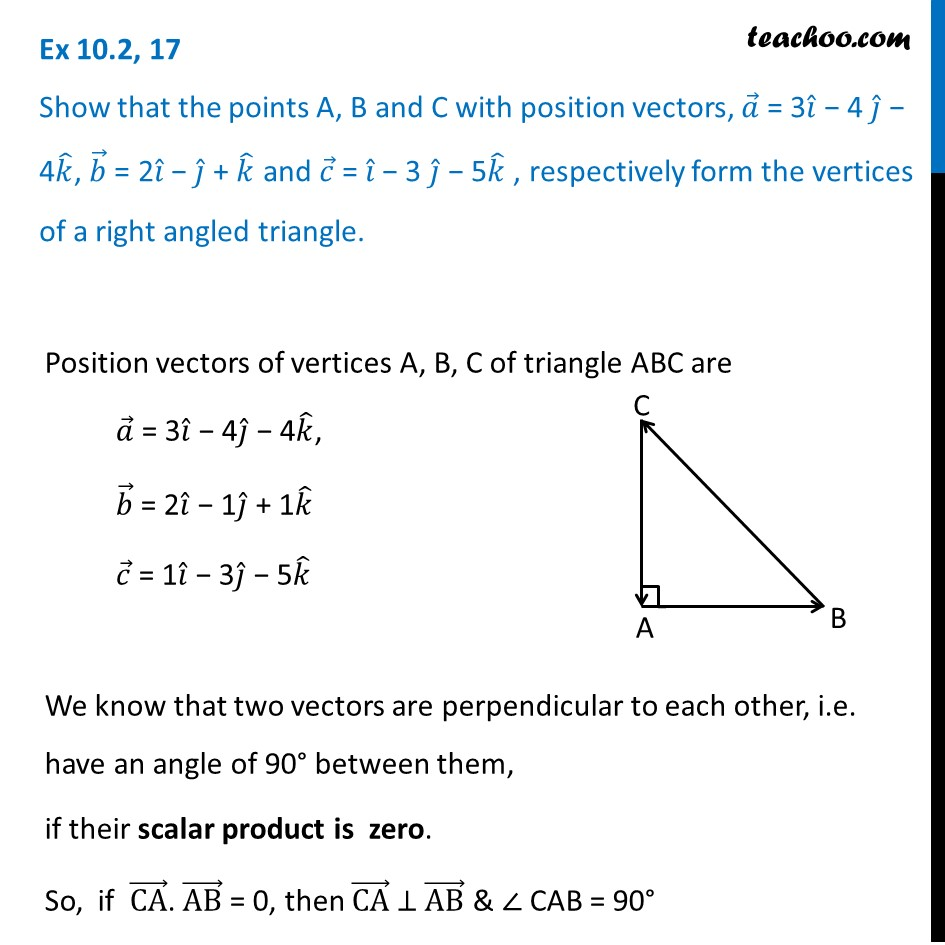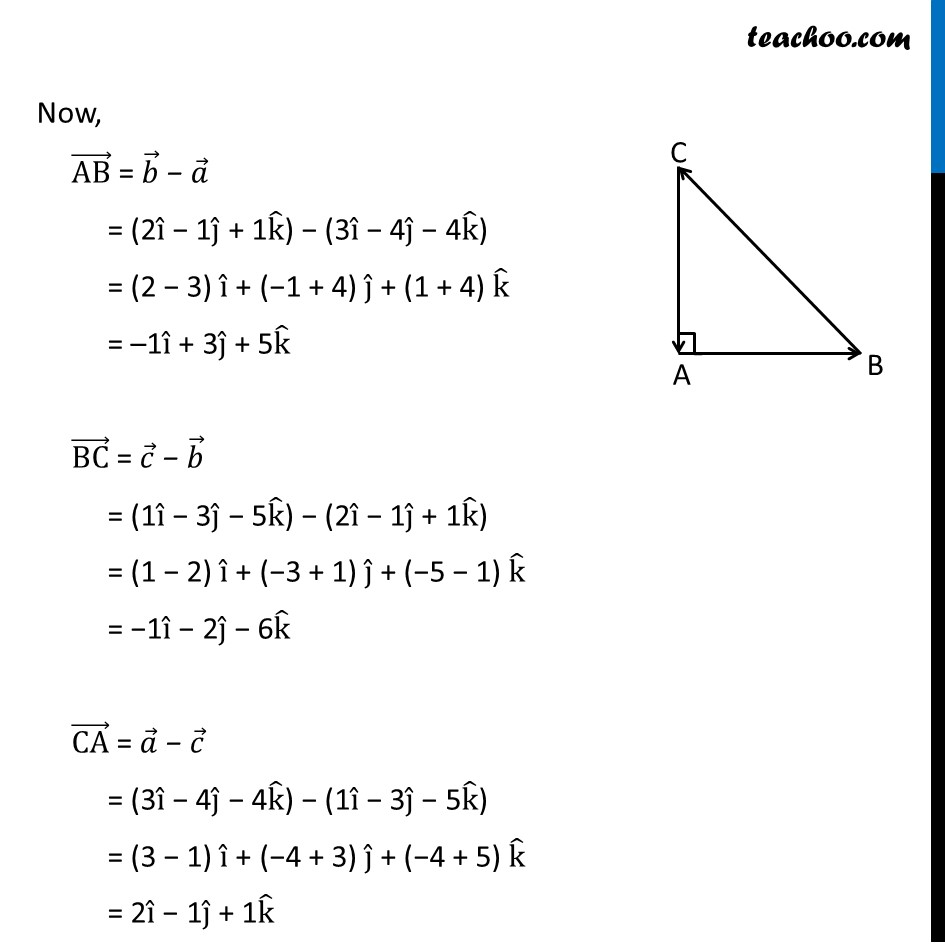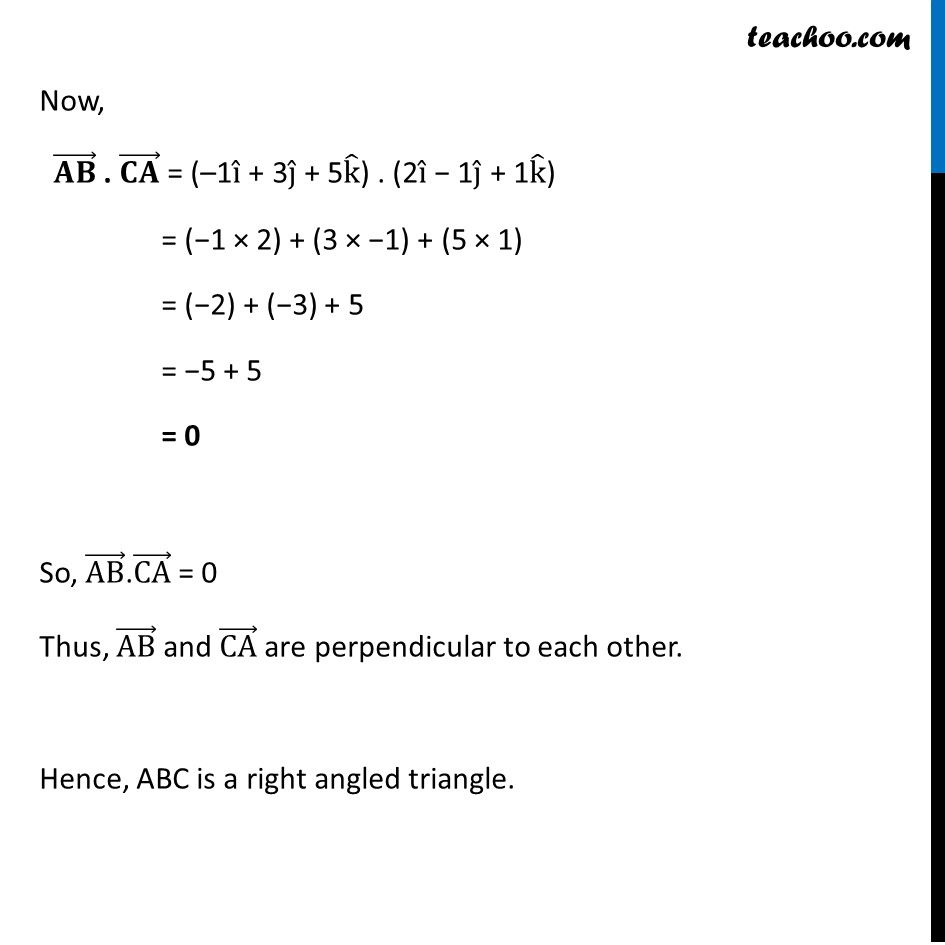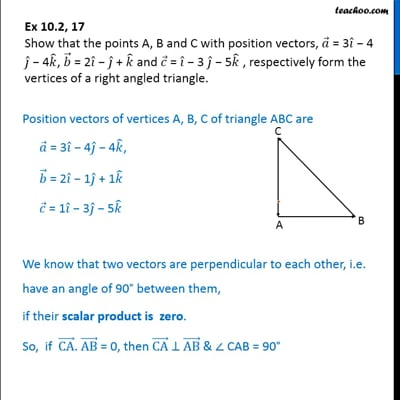Right Angled triangle

Chapter 10 Class 12 Vector Algebra
Concept wiseThis video is only available for Teachoo black users

Introducing your new favourite teacher - Teachoo Black, at only ₹83 per month

### Transcript

Ex 10.2, 17 Show that the points A, B and C with position vectors, 𝑎 ⃗ = 3𝑖 ̂ − 4 𝑗 ̂ − 4𝑘 ̂, 𝑏 ⃗ = 2𝑖 ̂ − 𝑗 ̂ + 𝑘 ̂ and 𝑐 ⃗ = 𝑖 ̂ − 3 𝑗 ̂ − 5𝑘 ̂ , respectively form the vertices of a right angled triangle. Position vectors of vertices A, B, C of triangle ABC are 𝑎 ⃗ = 3𝑖 ̂ − 4𝑗 ̂ − 4𝑘 ̂, 𝑏 ⃗ = 2𝑖 ̂ − 1𝑗 ̂ + 1𝑘 ̂ 𝑐 ⃗ = 1𝑖 ̂ − 3𝑗 ̂ − 5𝑘 ̂ We know that two vectors are perpendicular to each other, i.e. have an angle of 90° between them, if their scalar product is zero. So, if (CA) ⃗. (AB) ⃗ = 0, then (CA) ⃗ ⊥ (AB) ⃗ & ∠ CAB = 90° Now, (AB) ⃗ = 𝑏 ⃗ − 𝑎 ⃗ = (2i ̂ − 1j ̂ + 1k ̂) − (3i ̂ − 4j ̂ − 4k ̂) = (2 − 3) i ̂ + (−1 + 4) j ̂ + (1 + 4) k ̂ = –1i ̂ + 3j ̂ + 5k ̂ (BC) ⃗ = 𝑐 ⃗ − 𝑏 ⃗ = (1i ̂ − 3j ̂ − 5k ̂) − (2i ̂ − 1j ̂ + 1k ̂) = (1 − 2) i ̂ + (−3 + 1) j ̂ + (−5 − 1) k ̂ = −1i ̂ − 2j ̂ − 6k ̂ (CA) ⃗ = 𝑎 ⃗ − 𝑐 ⃗ = (3i ̂ − 4j ̂ − 4k ̂) − (1i ̂ − 3j ̂ − 5k ̂) = (3 − 1) i ̂ + (−4 + 3) j ̂ + (−4 + 5) k ̂ = 2i ̂ − 1j ̂ + 1k ̂ Now, (𝐀𝐁) ⃗ . (𝐂𝐀) ⃗ = (–1i ̂ + 3j ̂ + 5k ̂) . (2i ̂ − 1j ̂ + 1k ̂) = (−1 × 2) + (3 × −1) + (5 × 1) = (−2) + (−3) + 5 = −5 + 5 = 0 So, (AB) ⃗.(CA) ⃗ = 0 Thus, (AB) ⃗ and (CA) ⃗ are perpendicular to each other. Hence, ABC is a right angled triangle.